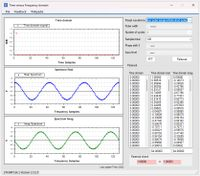# Time, Frequency and Parseval theorem demonstrator.

With this tool the relationship between time and frequency domain is shown. In the example blow you see a time shifted (phase shift = 3) Dirac pulse (Infinite high and infinite short pulse in the time domain at T=0) causing the real and imaginary spectrum to be two sine waves.

If you think the other way around, i.e. the sine-cosine waves being real-imag time domain components, the a sine wave in the time domain causes a single spectral line in the frequency domain (in this example the dot in the time domain graph).

At present the calculator input is time domain, future updates will also have entry in the frequency domain.

The Parseval theorem:

This mathematical theorem states that the energy in both domains must be the same.>100 RF Calculators
WinRFCalc, the best RF calculator toolbox for Windows